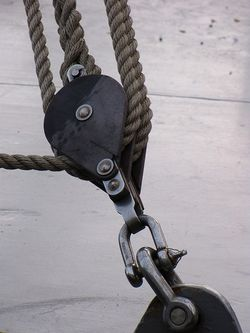# Pulley

In other languages: FrançaisFigure 1: A pulley being used on a boat, consisting of a metal wheel and a rope.

A pulley is a simple machine that can change the magnitude and direction of an applied force in the process of doing work. It consists of a cable which is attached to a wheel and axle, and is often used to lift/transport objects. When referred to in this context, these objects that are being moved are generally known as the load. Figure 1 shows a simple pulley but pulleys often work together in systems; figure 2 shows examples of pulley systems.

Pulleys use the concept of mechanical advantage, which shows how much an applied force is a multiplied (and the distance that force moves decreases). For pulleys, the ideal mechanical advantage is

, where is the number of fixed-axis pulleys.

For example, if the mechanical advantage of a pulley system is 3, the pulley system has 3 fixed pulleys acting on an object, therefore reducing the force that needs to be applied by a factor of 3. Due to the rules of conservation of energy however, the distance that this force needs to act over is three times the distance that the load will actually move. This comes from the idea that work is force times distance, this means that Win = Wout.

The pulleys in Figure 2 illustrate what creates this mechanical advantage; the tension of the ropes. The load is pulling down on the centre of the pulley which is supported by cables or ropes. Therefore the force of the load is distributed evenly among the cables. For the case of two pulleys (the second pulley in Figure 3), the force of this object is distributed to only two cables, therefore yielding a mechanical advantage of 2. It can be seen from the other systems that this principle applies more so as more pulleys are added.

It is important to understand that although the force is decreased using these systems, this doesn't change the amount of work done. Another important thing to remember is that these are ideal systems; the cable and pulleys masses are neglected, and there are only conservative forces acting on the system. In reality these objects have masses, and there are many energy losses due to friction and sound.

## Authors and Editors

Ethan Boechler, Jordan Hanania, Kailyn Stenhouse, Jason Donev
Last updated: September 27, 2021
Get Citation